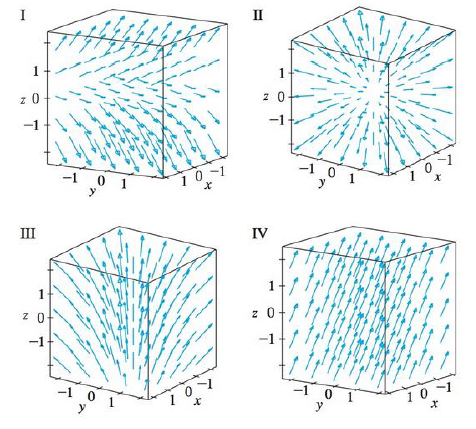Chapter 16.1, Problem 16E

Chapter
Section
Textbook Problem

Match the vector fields F on ℝ3 with the plots labeled I-IV. Give reasons for your choices.16. F(x, y, z) = i + 2 j + z kTo determine

To match: The vector field F(x,y,z)=i+2j+zk with the plots labeled as I-IV.

Explanation

Given data:

F(x,y,z)=i+2j+zk=1,2,z

Formula used:

Consider a three-dimensional vector F=x,y,z .

Write the expression for length of the three dimensional vector.

|F(x,y,z)|=x2+y2+z2 (1)

Find the length of F(x,y,z) using equation (1)

Still sussing out bartleby?

Check out a sample textbook solution.

See a sample solution

The Solution to Your Study Problems

Bartleby provides explanations to thousands of textbook problems written by our experts, many with advanced degrees!

Get Started

In Exercises 516, evaluate the given quantity. log5125

Finite Mathematics and Applied Calculus (MindTap Course List)

In Exercises 75-98, perform the indicated operations and/or simplify each expression. 92. (3x + 2)(2 3x)

Applied Calculus for the Managerial, Life, and Social Sciences: A Brief Approach

Solve the equations in problems 1-6 6.

Mathematical Applications for the Management, Life, and Social Sciences

True or False: is a convergent series.

Study Guide for Stewart's Multivariable Calculus, 8th

The average value of f(x) = 3x2 + 1 on the interval [2, 4] is: a) 29 b) 66 c) 58 d) 36

Study Guide for Stewart's Single Variable Calculus: Early Transcendentals, 8th